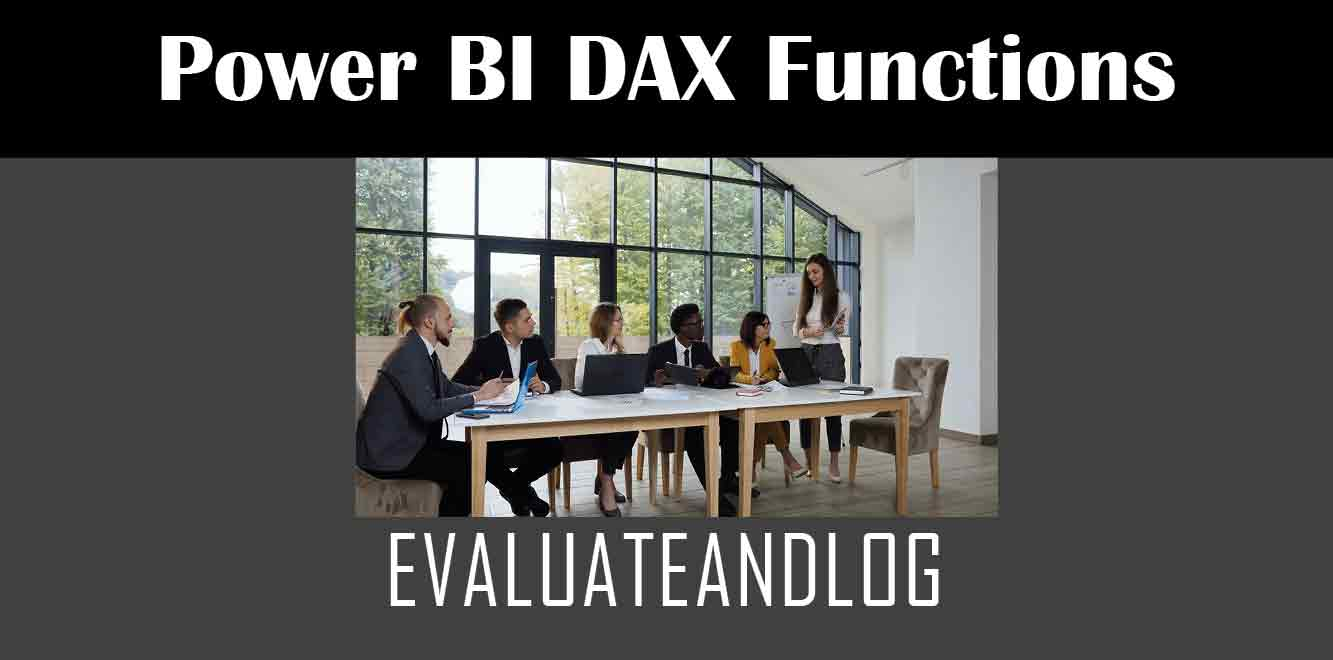# EVALUATEANDLOG

## YIn this article, we’ll explore the EVALUATEANDLOG function in detail, including what it is, how it works, and some practical examples of how to use it.

## What is EVALUATEANDLOG?

EVALUATEANDLOG is a DAX function that allows you to evaluate an expression and log the result to a table. The function returns a table that contains a single row and column, which shows the result of the expression you evaluated.

The syntax for EVALUATEANDLOG is as follows:

EVALUATEANDLOG ( ❰expression❱, ❰table name❱, ❰column name❱ )

``` Where: - `❰expression❱` is the DAX expression you want to evaluate. - `❰table name❱` is the name of the table where you want to log the result. - `❰column name❱` is the name of the column where you want to log the result. How does EVALUATEANDLOG work? To understand how EVALUATEANDLOG works, let's take a look at a simple example. Suppose you have a table called Sales, which contains data on the sales of various products. You want to create a new table that shows the total sales for each product category. Here's how you can use EVALUATEANDLOG to do this: ```

EVALUATEANDLOG (

SUMMARIZE (

Sales,

Sales[Product Category],

“Total Sales”, SUM ( Sales[Sales Amount] )

),

“Sales by Product Category”,

“Total Sales”

)

``` In this example, we're using SUMMARIZE to group the Sales table by product category and calculate the total sales for each category. We then use EVALUATEANDLOG to evaluate this expression and log the result to a new table called Sales by Product Category. The result of this function will be a table with two columns: Product Category and Total Sales. Practical examples of using EVALUATEANDLOG Let's take a look at some practical examples of how EVALUATEANDLOG can be used in Power BI. Example 1: Logging the results of a measure Suppose you have a measure called Total Sales, which calculates the total sales for a given period. You want to log the result of this measure to a table called Sales Log. Here's how you can do this using EVALUATEANDLOG: ```

EVALUATEANDLOG (

CALCULATE ( [Total Sales], Dates[Month] = “January” ),

“Sales Log”,

“Total Sales”

)

``` In this example, we're using CALCULATE to calculate the Total Sales measure for the month of January. We then use EVALUATEANDLOG to evaluate this expression and log the result to a new table called Sales Log. The result of this function will be a table with a single row and column, which shows the total sales for January. Example 2: Logging the results of a complex expression Suppose you have a complex DAX expression that calculates the revenue for a given period. You want to log the result of this expression to a table called Revenue Log. Here's how you can do this using EVALUATEANDLOG: ```

EVALUATEANDLOG (

CALCULATE (

SUM ( Sales[Sales Amount] ) * 0.8 + SUM ( Returns[Return Amount] ),

Dates[Month] = “January”

),

“Revenue Log”,

“Revenue”

)

``` In this example, we're using CALCULATE to calculate a complex expression that calculates the revenue for the month of January. We then use EVALUATEANDLOG to evaluate this expression and log the result to a new table called Revenue Log. The result of this function will be a table with a single row and column, which shows the revenue for January. In conclusion, EVALUATEANDLOG is a powerful DAX function that allows you to evaluate an expression and log the result to a table. By using this function, you can easily track the results of your calculations and analyze your data more effectively. In this article, we've explored what EVALUATEANDLOG is, how it works, and some practical examples of how to use it. With this knowledge, you should be able to use EVALUATEANDLOG to enhance your Power BI reports and make your data more useful and actionable. Power BI DAX Training Courses by G Com Solutions (0800 998 9248)Power BI DAX Intensive Training Course £1,050.00 – £26,550.00 Select optionsContinue Loading DonePower BI DAX Introduction £395.00 – £9,750.00 Select optionsContinue Loading DonePower BI DAX Intermediate £395.00 – £9,750.00 Select optionsContinue Loading DonePower BI DAX Advanced £395.00 – £9,750.00 Select optionsContinue Loading Done Upcoming Courses Contact Us Subject Your Name (required) Company/Organisation Email (required) Telephone Training Course(s) Power BI Intensive TrainingPower BI introduction Power BI IntermediatePower BI AdvancedDAXPower Query MPower BI CertificationPower BI AdministrationPower PlatformPower AutomatePower AppsOTHER Your Message Upload Example Document(s) (Zip multiple files) ```
``` ```
``` ```
``` ```
``` ```
``` ```